International
Tables for
Crystallography
Volume B
Reciprocal space
Edited by U. Shmueli

International Tables for Crystallography (2010). Vol. B, ch. 2.5, pp. 361-362   | 1 | 2 |

## Section 2.5.5.2. Thin weak phase objects at optimal defocus

B. K. Vainshteinc

#### 2.5.5.2. Thin weak phase objects at optimal defocus

| top | pdf |

The intensity distribution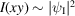of an electron wave in the image plane depends not only on the coherent and inelastic scattering, but also on the instrumental functions. The electron wave transmitted through an object interacts with the electrostatic potentialwhich is produced by the nuclei charges and the electronic shells of the atoms. The scattering and absorption of electrons depend on the structure and thickness of a specimen, and the atomic numbers of the atoms of which it is composed. If an object with the three-dimensional distribution of potentialis sufficiently thin, then the interaction of a plane electron wavewith it can be described as the interaction with a two-dimensional distribution of potential projection,where b is the specimen thickness. It should be noted that, unlike the three-dimensional function of potentialwith dimension, the two-dimensional function of potential projectionhas the potential-length dimensionwhich, formally, coincides with the charge dimension. The transmission function, in the general case, has the form(2.5.2.42), and for weak phase objects the approximationis valid.

In the back focal plane of the objective lens the wave has the form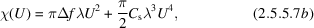where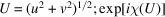is the Scherzer phase function (Scherzer, 1949) of an objective lens (Fig. 2.5.5.1),is the aperture function,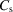the spherical aberration coefficient, and Δf the defocus value [(2.5.2.32)–(2.5.2.35)].Figure 2.5.5.1 | top | pdf |The χ function and two components of the Scherzer phase function sinand cos.

The bright-field image intensity (in object coordinates) is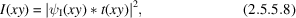where. The phase function (2.5.5.7)depends on defocus, and for a weak phase object (Cowley, 1981)where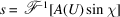, which includes only an imaginary part of function (2.5.5.6). While selecting defocus in such a way that under the Scherzer defocus conditions [(2.5.2.44), (2.5.2.45)], one could obtainIn this very simple case the image reflects directly the structure of the object – the two-dimensional distribution of the projection of the potential convoluted with the spread function. In this case, no image restoration is necessary. Contrast reversal may be achieved by a change of defocus.

At high resolution, this method enables one to obtain an image of projections of the atomic structure of crystals and defects in the atomic arrangement – vacancies, replacements by foreign atoms, amorphous structures and so on; at resolution worse than atomic one obtains images of dislocations as continuous lines, inserted phases, inclusions etc. (Cowley, 1981). It is also possible to obtain images of thin biological crystals, individual molecules, biological macromolecules and their associations.

Image restoration. In the case just considered (2.5.5.10), the projection of potential, convoluted with the spread function, can be directly observed. In the general case (2.5.5.9), when the aperture becomes larger, the contribution to image formation is made by large values of spatial frequencies U, in which the function sin χ oscillates, changing its sign. Naturally, this distorts the image just in the region of appropriate high resolution. However, if one knows the form of the function sin χ (2.5.5.7), the true functioncan be restored.

This could be carried out experimentally if one were to place in the back focal plane of an objective lens a zone plate transmitting only one-sign regions of sin χ (Hoppe, 1971). In this case, the information onis partly lost, but not distorted. To perform such a filtration in an electron microscope is a rather complicated task.

Another method is used (Erickson & Klug, 1971). It consists of a Fourier transformationof the measured intensity distribution TQ (2.5.5.6)and division of this transform, according to (2.5.5.7a,b), by the phase function sin χ. This givesThen, the new Fourier transformationyields (in the weak-phase-object approximation) the true distributionThe function sin χ depending on defocus Δf should be known to perform this procedure. The transfer function can also be found from an electron micrograph (Thon, 1966). It manifests itself in a circular image intensity modulation of an amorphous substrate or, if the specimen is crystalline, in the `noise' component of the image. The analogue method (optical Fourier transformation for obtaining the image) can be used (optical diffraction, see below); digitization and Fourier transformation can also be applied (Hoppe et al., 1973).

The thin crystalline specimen implies that in the back focal objective lens plane the discrete kinematic amplitudesare arranged and, by the above method, they are corrected and released from phase distortions introduced by the function sin χ (see below) (Unwin & Henderson, 1975).

For the three-dimensional reconstruction (see Section 2.5.6) it is necessary to have the projections of potential of the specimen tilted at different angles α to the beam direction (normal beam incidence corresponds to). In this case, the defocus Δf changes linearly with increase of the distance l of specimen points from the rotation axis. Following the above procedure for passing on to reciprocal space and correction of sin χ, one can find(Henderson & Unwin, 1975).

### References

Cowley, J. M. (1981). Diffraction Physics, 2nd ed. Amsterdam: North-Holland.
Erickson, H. P. & Klug, A. (1971). Measurements and compensation of defocusing and aberrations by Fourier processing of electron micrographs. Philos. Trans. R. Soc. London Ser. B, 261, 105–118.
Henderson, R. & Unwin, P. N. T. (1975). Three-dimensional model of purple membrane obtained by electron microscopy. Nature (London), 257, 28–32.
Hoppe, W. (1971). Use of zone correction plate and other techniques for structure determination of aperiodic objects at atomic resolution using a conventional electron microscope. Philos. Trans. R. Soc. London Ser. B, 261, 71–94.
Hoppe, W., Bussler, P., Feltynowski, A., Hunsmann, N. & Hirt, A. (1973). Some experience with computerized image reconstruction methods. In Image Processing and Computer-Aided Design in Electron Optics, edited by R. W. Hawkes, pp. 92–126. London: Academic Press.
Scherzer, O. (1949). The theoretical resolution limit of the electron microscope. J. Appl. Phys. 20, 20–29.
Thon, F. (1966). On the defocusing dependence of phase contrast in electron microscopical images. Z. Naturforsch. Teil A, 21, 476–478.
Unwin, P. N. T. & Henderson, R. (1975). Molecular structure determination by electron microscopy of unstained crystalline specimens. J. Mol. Biol. 94, 425–440.